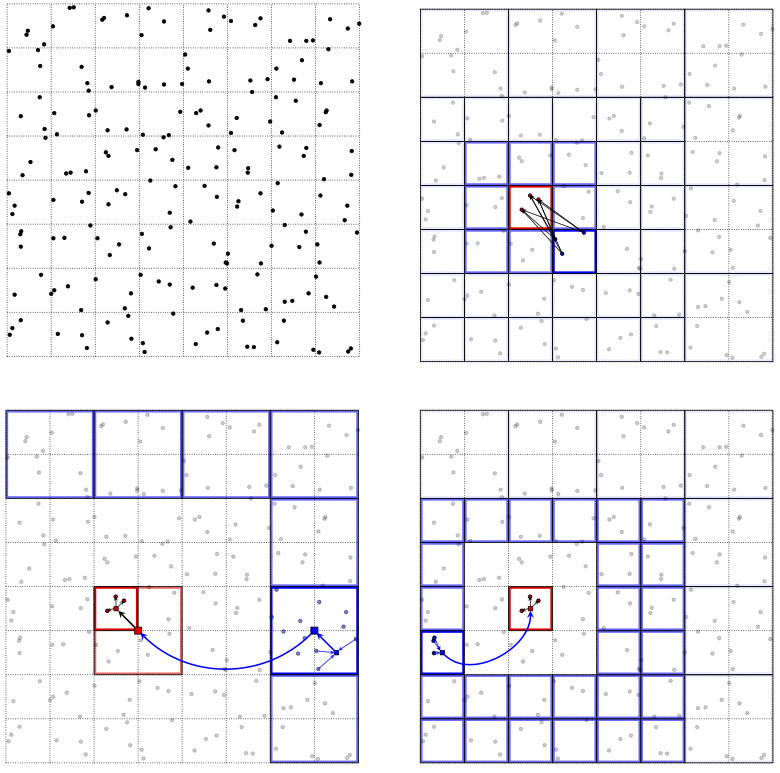# Fast multipole methods in two dimensions (fmm2d)¶fmm2d is a set of libraries to compute N-body interactions governed by the Laplace and Helmholtz equations, to a specified precision, in three dimensions, on a multi-core shared-memory machine. The library is written in Fortran, wrappers will soon be available for C, MATLAB, and Python. As an example, given $$M$$ arbitrary points $$y_j \in \mathbb{R}^{2}$$ with corresponding real numbers $$c_j$$, and $$N$$ arbitrary points $$x_{j} \in \mathbb{R}^{2}$$, the Laplace FMM evaluates the $$N$$ real numbers

(1)$u_{\ell} = \sum_{j=1}^M c_j\log{\| x_{\ell} - y_{j}\|} ~, \qquad \mbox{ for } \; \ell=1,2,\ldots N ~.$

The $$y_j$$ can be interpreted as source locations, $$c_j$$ as charge strengths, and $$u_{\ell}$$ as the resulting potential at target location $$x_{\ell}$$.

Such N-body interactions are needed in many applications in science and engineering, including molecular dynamics, astrophysics, rheology, and the numerical solution of partial differential equations. The naive CPU effort to evaluate (1) is $$O(NM)$$. The FMM approximates (1) to a requested relative precision $$\epsilon$$ with linear effort $$O((M+N) \log (1/\epsilon))$$.

The FMM relies on compressing the interactions between well-separated clusters of source and target points at a hierarchy of scales using analytic outgoing, incoming, and plane-wave expansions of the interaction kernel and associated translation operators. This library is an improved version of the FMMLIB2D software, Copyright (C) 2010-2012: Leslie Greengard and Zydrunas Gimbutas, released under the BSD license. The major improvements are the following:

• Vectorization of the FMM, to apply the same kernel with the same source and target locations to multiple strength vectors

• A redesign of the adaptive tree data structure

• Diagonal form translation operators for high frequency Helmholtz problems

Note

For very small repeated problems (less than 1000 input and output points), users should also consider dense matrix-matrix multiplication using BLAS3 (eg DGEMM,ZGEMM).